# Chew-Goldberger-Low Forms

As is well known, the smallness of the toroidal magnetization parameter,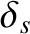, ensures that the dominant parallel viscosity tensors,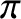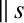and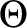, take the so-called Chew-Goldberger-Low forms [11,29]: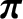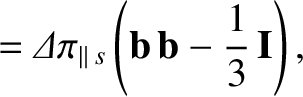(2.152)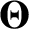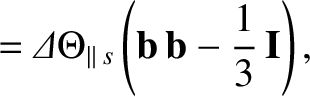(2.153)

where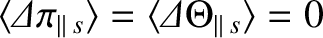. Incidentally, it is clear from Equation (2.60) that the parallel viscosity tensor in the classical closure scheme does indeed take this form.

Now, iftakes the Chew-Goldberger-Low form then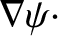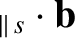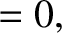(2.154)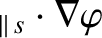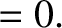(2.155)

These results follow because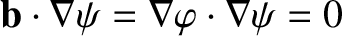.

Furthermore,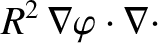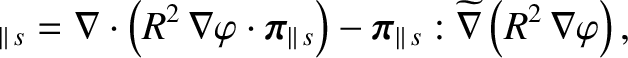(2.156)

where, in Cartesian coordinates,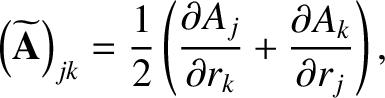(2.157)

and use has been made of the fact thatis a symmetric tensor. In fact, it can be shown that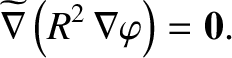(2.158)

Hence, we deduce that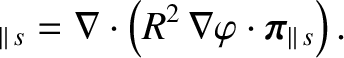(2.159)

Making use of Equation (2.150), we obtain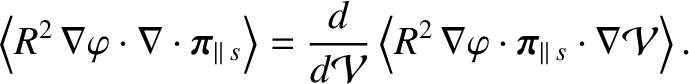(2.160)

However, iftakes the Chew-Goldberger-Low form then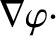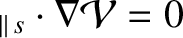, because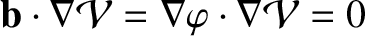. It follows that [32,34]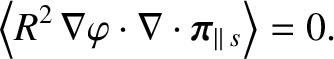(2.161)

Likewise, iftakes the Chew-Goldberger-Low form then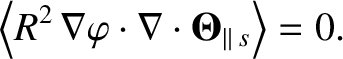(2.162)